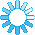Machine LearningWelcome to Machine Learning section of C# Corner. In this section, you will find various Machine Learning related source code samples, articles, tutorials, and tips.## RECENT POSTS

• ### Understanding K-Means Clustering using Python the easy way

K-means clustering is a simple unsupervised learning algorithm that is used to solve clustering problems. It follows a simple procedure of classifying a given data set into a number of clusters, de...
Jan 13, 2020
• ### Chronic Kidney Disease Prediction In ASP.NET Core Using ML.NET

In this article, we'll learn how ML.NET framework is used to build a Chronic Kidney Disease Prediction machine learning model and integrate them into ASP.NET Core MVC application.
Dec 30, 2019
• ### Getting Started With Azure Jupyter Notebook📋 With Azure ML Studio (Preview)

In this article, we will focus on how to get started Azure ML Notebook. Moreover, we will discuss why we use Azure ML services with a simple demo.
Dec 30, 2019
• ### Build A Machine Learning Model With ML.NET CLI

In this article, we will learn how to build a Machine Learning model in ML.NET Framework using ML.NET CLI.
Dec 30, 2019
• ### Getting Started With☁️Azure Automated Machine Learning🚀

In this article, we will focus on what Azure automated Machine Learning is, why we are using it and how we handle it with a simple demo.
Dec 27, 2019
• ### Naive Bayes using Python- A Beginner's Tutorial

Naïve Bayes classifiers are a family of simple "probabilistic classifiers" based on applying Bayes' theorem with strong (naïve) independence assumptions between the features...
Dec 25, 2019
• ### KNN- K-Nearest Neighbors using Python

The k-nearest neighbors (KNN) algorithm is a simple, supervised machine learning algorithm that can be used to solve both classification and regression problems. It's easy to implement and unde...
Dec 25, 2019
• ### Implementation of Decision Trees In Python

Learn basics of decisions trees and their roles in computer algorithms and how decision trees are used in Python and machine learning.
Dec 25, 2019
• ### Logistic Regression using Python

Logistic regression is a statistical model that in its basic form uses a logistic function to model a binary dependent variable, although many more complex extensions exist. In regression analysis,...
Dec 25, 2019
• ### Understanding Linear Regression using Python

In statistics, linear regression is a linear approach to modeling the relationship between a scalar response and one or more explanatory variables. The case of one explanatory variable is called a ...
Dec 25, 2019
• ### Multiple Linear Regression using Python

Multiple linear regression (MLR), also known simply as multiple regression, is a statistical technique that uses several explanatory variables to predict the outcome of a response variable. In this...
Dec 25, 2019
• ### Data Science- A Beginner's Tutorial

This is the twelfth tutorial in this series. Data science is a multi-disciplinary field that uses scientific methods, processes, algorithms, and systems to extract knowledge and insights from struc...
Dec 24, 2019
• ### Car Evaluation In ASP.NET Core Using ML.NET

In this article, we'll learn how ML.NET framework is used to build, evaluate, train and consume the Car evaluation machine learning model and integrate them into ASP.NET Core MVC application.
Dec 23, 2019
• ### Challenges In Machine Learning (Training And Validation) - Part Three

This is the last part of the three series article - "Challenges in Machine Learning" that covers manipulating the data to get the best accuracy of the of machine learning model.
Dec 11, 2019
• ### Best Python Machine Learning Libraries

Python and machine learning go hand and hand. Here is a list of most popular machine learning libraries for Python.
Dec 11, 2019

Dec 04, 2019
• ### Challenges In Machine Learning - Part One

This article covers major algorithmic challenges faced in a machine learning project.
Dec 03, 2019
• ### ONNX Explained

This post explains the ONNX utility
Dec 01, 2019
• ### Python Basics

Python is a scripting language, which is an open-source language. It finds its use as one of the most powerful machine learning languages. Here, we will take an overview of Python language
Nov 27, 2019
• ### Batch And Online Machine Learning

There are so many ways to classify machine learning systems, and in this article, we are going to look at classification based on whether or not the machine system can learn incrementlly on the fl...
Nov 26, 2019
• ### How To Do Binary Classification in ASP.Net Core Using ML.Net

In this article, we will see binary classification in ASP.Net Core using ML.Net.
Oct 30, 2019
• ### All about Deep Learning Tutorial

This is the eleventh tutorial in the series. In this tutorial, we will be studying Deep Learning. Deep learning is a subset of machine learning in artificial intelligence (AI) that has networks cap...
Oct 23, 2019
• ### Topcoder Announces New Data Science And AI Features

Topcoder adds new data science and AI features to the Topcoder Platform to Advance Enterprise Analytics.
Oct 22, 2019
• ### All about Machine Learning

This is the tenth tutorial in the series. In this tutorial, we will be studying Machine Learning. Machine learning is the scientific study of algorithms and statistical models that computer systems...
Oct 22, 2019
• ### Artificial Intelligence - a Beginner's Tutorial

This is the ninth tutorial in the series. In this tutorial, we will be studying Artificial Intelligence and its functionalities. Artificial intelligence (AI) is the simulation of human intelligence...
Oct 21, 2019
• View More